Courses
Courses for Kids
Free study material
Offline Centres
More

# LCM of 50 and 70Last updated date: 02nd Dec 2023
Total views: 59.1k
Views today: 0.59k## Introduction to the Concept of LCM

The full form of LCM is the least common multiple. At least 2 numbers need to find the least common multiple. The least common multiple of two numbers or more than two is the least number that divides the numbers evenly or without leaving any remainder. In other words, the LCM of two numbers or more than two numbers is the least common multiple of the numbers. There is a unique LCM of any two numbers.

## Definition of Least Common Multiple

Given two numbers p and q, the least common multiple (LCM) of p and q is the least number that is divisible by both p and q, i.e., it is the least multiple of p and q.

LCM of p and q is denoted by: lcm (p,q).

Let us suppose p = 10 and q = 15. Multiples of p are 10, 20, 30, 40, 50, 60, … and Multiples of q are 15, 30, 45, 60, 75, 90, …. We can see clearly that the common multiples of p and q are 30, 60, …. Out of these, the smallest number is 30. So, the least common multiple of 10 and 15 is 30.

## Common Prime Factors of 50 and 70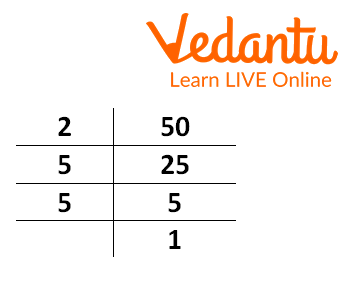Prime factorization of 50

Prime factorization of 50 is $2×5×5$$2 \times 5 \times 5$.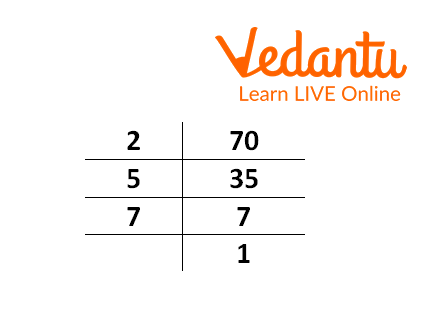Prime factorization of 70

Prime factorization of 70 is $2×5×7$$2 \times 5 \times 7$.

The common prime factors of 50 and 70 are 2 and 5.

## Methods to find LCM of 50 and 70

There are 3 methods to find the LCM of two numbers:

• Common Multiples Method

• Prime Factorization Method

• Division Method

## LCM by Common Multiples Method

Given two numbers a and b. In this method, we write the multiples of a and b separately and take out the common multiples of a and b. Then we find the smallest of those multiples and label it as the Least Common Multiple (LCM) of a and b.

Since we need to find the lcm of 50 and 70, take a=50, b=70.

The method is illustrated as:

Multiples of a: 50, 100, 150, 200, 250, 300, 350, 400, 450, 500, 550, 600, 650, 700, …

Multiples of b: 70, 140, 210, 280, 350, 420, 490, 560, 630, 700, 770, 840, 910, 980, …

Common Multiples of a and b: 350, 700, 1050, …

Least Common Multiple lcm (50,70) = 350.

## LCM by Prime Factorisation Method

Consider the same two numbers, a and b. In this method, we write the prime factorisation of both numbers. For writing prime factorization, we start from the smallest prime number, i.e., 2, and start dividing the number at the right to get 1. If 2  doesn’t divide the number, we can take the next prime number.

Then we take the common factors in the prime factorization of both and write them in multiplication form considering them as one (For example, if ${2}^{2}$$2^2$ is present in both the prime factorizations, we write ${2}^{2}$$2^2$, not ${2}^{2}×{2}^{2}$$2^2\times2^2$). Then we write the remaining factors also in this multiplication.

We need to find what is the LCM of 50 and 70 by prime factorisation method, so, take a=50, b=70.

## The method is illustrated here:

1. ### Prime Factorisation of 50Prime factorization of 50

Prime Factorization of $50=2×{5}^{2}$$50 = 2\times5^2$

1. ### Prime Factorization of 70Prime factorization of 70

Prime factorisation of 70$=2×5×7$$= 2\times5\times7$

Common Prime Factors of 50 and 70 = 2,5

So, LCM of 50 and 70 $=2×5×5×7$$= 2\times5\times5\times7$ (Writing the common factors once and non-common factors also)

$Lcm\left(50,70\right)=2×{5}^{2}×7=2×25×7=350$$Lcm (50,70) = 2\times5^2\times7 = 2\times25\times7 = 350$

Least Common Multiple lcm (50,70) = 350.

## LCM by Division Method

Consider the same numbers a and b. In the division method, we write both numbers together. Then we start from the smallest prime number, i.e., 2, and check whether 2 divides the numbers. If yes, then we divide both numbers by 2 and write the quotients in the right column.

If 2 divides only one of them, we divide that number by 2, write its quotient, and write down the other number without any change. This way, we change the primes and continue until we get both numbers 1 at the right. Then we write the numbers obtained on the left-hand side in multiplication form. This is the prime factorisation of the LCM of both numbers.

We need to find the lcm of 50 and 70 $⇒a=50,b=70$$\Rightarrow a=50, b=70$

The division method is given here: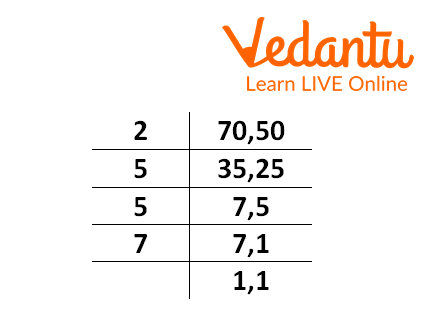Prime factorization of 50 and 70

Lcm of 50 and 70 $=2×5×5×7$$= 2\times5\times5\times7$

Lcm (50,70) $=2×{5}^{2}×7=2×25×7=350$$= 2\times5^2\times7 = 2\times25\times7 = 350$

Least Common Multiple LCM (50,70) = 350.

## Interesting Facts

• If you know two numbers and their gcd (greatest common divisor), you can find their LCM by the formula:

$\text{ gcd} (a,b)\times \text{lcm} (a,b) = a\times b$

• LCM is very useful in daily life. During Diwali, you must have seen lights glowing alternatively with different timings. If we need to find how much time all the lights glow together, we find the LCM of timings of individual lights.

## Solved Questions

1. Find LCM of 20 and 30 using the division method.

Answer: We use the division method as: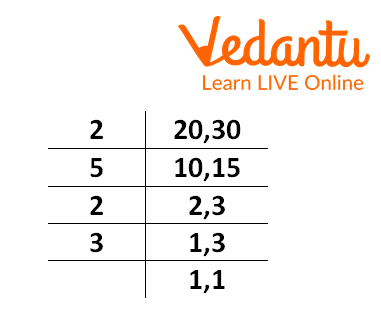Prime factorization of 20,30

Hence, LCM (20,30) $=2×2×3×5$$= 2\times2\times3\times5$

$={2}^{2}×3×5$$=2^2\times3\times5$

$=4×3×5$$=4\times3\times5$

=60

So, the LCM of 20 and 30 is 60.

2. Find the lowest number that is exactly divisible by 30 and 24.

Answer: We will find LCM of 30 and 24 to find the lowest number that is exactly divisible by 30 and 24.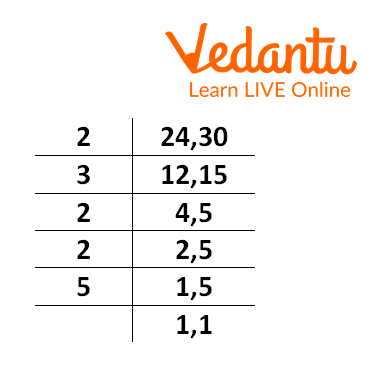Prime factorization 24 and 30

The LCM of 24 and 30 is ${2}^{3}×3×5=120$$2^3\times 3\times 5 = 120$

The lowest number that is exactly divisible by 30 and 24 is 120.

3. Find the lowest number which is less by 5 to be divided by 12, 24, and 30 exactly.

Answer: First, we will find the LCM of 12, 24, and 30.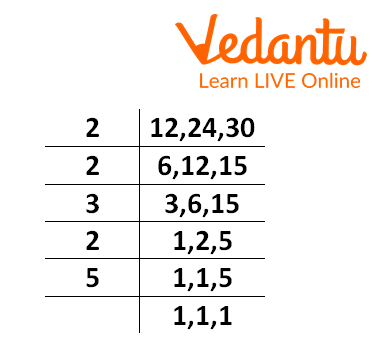Prime factorization 12, 24 and 30

The LCM of 12, 24 and 30 is ${2}^{3}×3×5=120$$2^3\times 3\times 5 = 120$

Now subtract 5 from the LCM to find the required answer:

120-5 = 115.

The required number is 115.

## Conclusion

• LCM of two numbers is their smallest common multiple.

• There are three methods to find the LCM, out of which the division method is the most commonly used method.

• LCM of more than two numbers can also be found using the same method.

## Practice Questions

1. Find the LCM of 50, 60, and 70 by using the prime factorization method.

## FAQs on LCM of 50 and 70

1. What is the difference between LCM and HCF?

The full form of LCM is Least common multiple. The full form of HCF is the highest common factor. HCF is the greatest common factor of two number or more numbers. LCM is the least common multiple of two numbers or more number.

2. If the HCF and LCM of two numbers are not equal then which is greater HCF and LCM of two numbers?

The factor of a number is always less than or equal to the number. Multiples of a number are greater than or equal to the number. Thus, HCF of two numbers is less than or equal to LCM. Since the HCF and LCM of the two numbers are not equal, the LCM of the two numbers is greater than HCF.

3. Is HCF always divisible by LCM?

Numbers are divisible by their HCF. LCM of numbers is a multiple of the given number. The factor of a number divides the multiples of numbers evenly. Thus, HCF is always divisible by LCM.